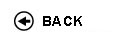Politecnico di Torino
04KWQLI, 04KWQJM, 04KWQLM, 04KWQLP, 04KWQLS, 04KWQLX, 04KWQLZ, 04KWQMA, 04KWQMB, 04KWQMC, 04KWQMH, 04KWQMK, 04KWQMO, 04KWQMQ, 04KWQNX, 04KWQOD, 04KWQPC, 04KWQPI, 04KWQPL
Mathematical analysis I
1st degree and Bachelor-level of the Bologna process in Automotive Engineering - Torino
1st degree and Bachelor-level of the Bologna process in Mechanical Engineering - Torino
1st degree and Bachelor-level of the Bologna process in Computer Engineering - Torino
Espandi...
 Teacher Status SSD Les Ex Lab Tut Years teaching Boieri Paolo 60 40 0 0 7 Boieri Paolo 60 40 0 0 7
 SSD CFU Activities Area context MAT/05 10 A - Di base Matematica, informatica e statistica
Esclusioni:
16ACF
 Subject fundamentals This course represents a bridge between high school and college ways of teaching and learning. The main goal of the course is to provide the students with tools to understand and elaborate logical arguments, from simple to more elaborate ones, and to introduce the elements of differential and integral calculus for functions of one variable with applications to ordinary differential equations of the first order and of the second order linear case. Expected learning outcomes Ability to follow a chain of logical arguments. Essentials of differential and integral calculus for functions of one variable. Computational ability. Prerequisites / Assumed knowledge Numbers, equations and inequalities, analytical geometry and trigonometry. First properties of elementary functions. Contents Preliminaries: sets and operations with sets. Numerical sets, maxima, minima and extrema. The completeness propertiy of real numbers and its consequences. Functions: surjectivity and injectivity; composition of functions, inverse functions. Functions of a real variable: elementary functions, monotone functions and inverse functions. (About 15 hours) Limits and continuity: theory of limits of functions and sequences. Continuous functions and related theorems. Order and comparison theorems. Algebra of limits. Indeterminate forms. Local comparison of functions. Landau symbols. Infinite and infinitesimal functions. Order of an infinity and of an infinitesimal, principal part with respect to a test function. Asymptotes. The number e. Fundamental trigonometric and exponential limits. Properties continuous functions on an interval: existence of zeros and of maxima and minima. (About 24 hours) Derivatives: geometrical and physical meaning. Computation of derivatives. List of fundamental derivatives. Derivatives and continuity. Lack of differentiability, extremal and critical points. Fermat Theorem. Properties of differentiable functions on an interval and fundamental theorems of differential calculus (Rolle and Lagrange theorems) and their consequences. De L'H�pital rule. Taylor formula and fundamental MacLaurin formulas. Use of Taylor expansions in the local analysis of functions: comparison, extrema and convexity. (About 23 hours) Primitives: rules of computation; primitives of rational functions. Indefinite integral. Riemann integral and its properties. Classes of integrable functions. Integral mean value. Fundamental theorem of integral calculus: relations between definite integrals and primitives. Improper integrals: definitions and convergence tests. (About 21 hours) Complex numbers and differential equations: algebraic and trigonometric forms of complex numbers. Real and imaginary parts, modulus and argument. Roots of complex numbers; Fundamental theorem of Algebra. Exponentials of complex numbers and Euler formulas. Ordinary differential equations: Cauchy problem. First order ordinary differential equations, linear or with separable variables. Second order linear differential equations with constant coefficients. (About 17 hours) Delivery modes 60 hours of theoretical classes and 40 hours of exercise classes. Texts, readings, handouts and other learning resources C. Canuto, A. Tabacco. Mathematical Analysis I. Springer-Verlag UTX, 2015. Assessment and grading criteria The exam consists of a test, a written part and, when required, of an oral part. The test (on computer, one hour long) consists of 20 multiple-choice questions of both theoretical and practical type. The maximum score achievable is 20. If the score is less than 10, the exam is failed, otherwise the student must take a written part of the exam (consisting of exercises and theory, one hour long). The sum of the scores of the test and of the written part is the final score of the exam, unless an oral part is required either by the teacher (or by the student, provided the final score is at least 18/30). In this case the final score will be based also on the oral part. Programma definitivo per l'A.A.2015/16